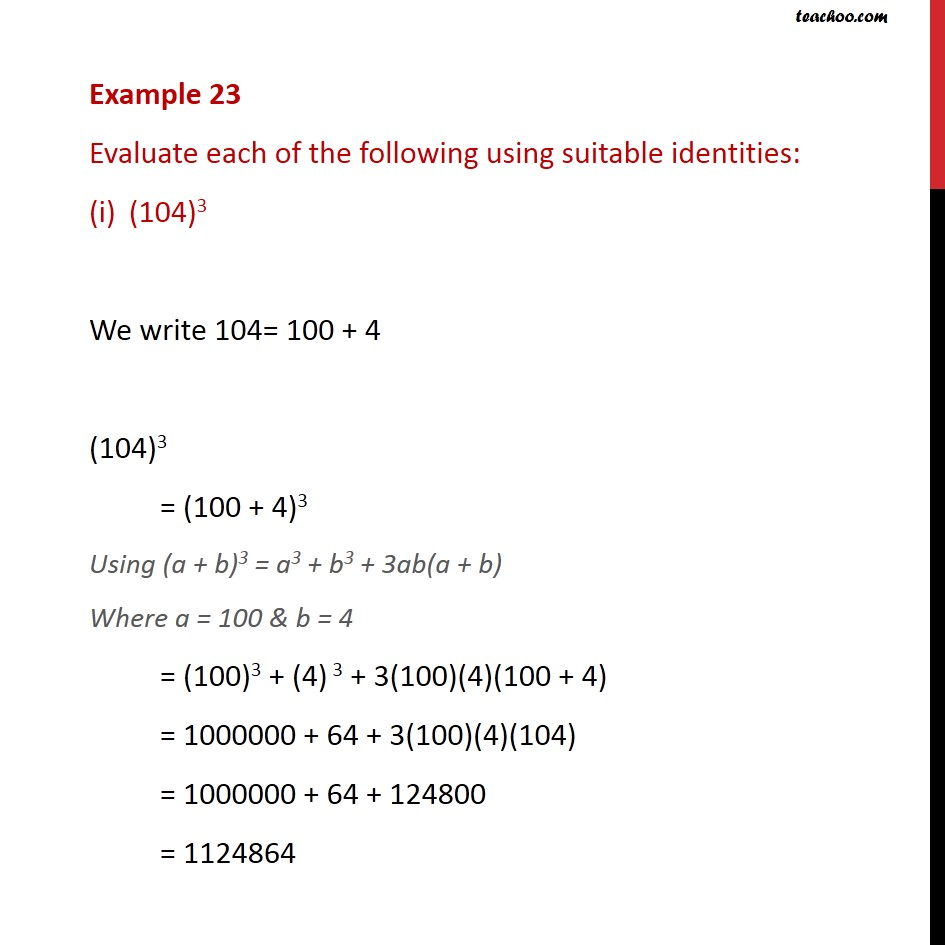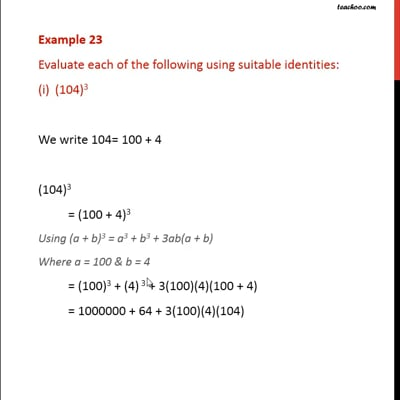Examples

Chapter 2 Class 9 Polynomials
Serial order wiseThis video is only available for Teachoo black users

Solve all your doubts with Teachoo Black (new monthly pack available now!)

### Transcript

Example 23 Evaluate each of the following using suitable identities: (104)3 We write 104= 100 + 4 (104)3 = (100 + 4)3 Using (a + b)3 = a3 + b3 + 3ab(a + b) Where a = 100 & b = 4 = (100)3 + (4) 3 + 3(100)(4)(100 + 4) = 1000000 + 64 + 3(100)(4)(104) = 1000000 + 64 + 124800 = 1124864